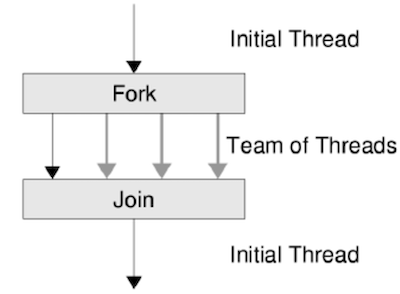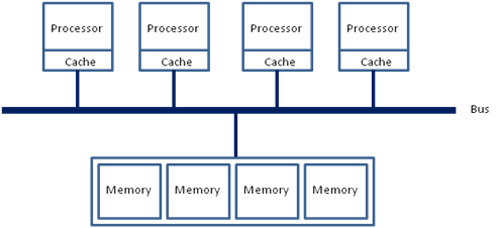# 共享内存多线程

## 1. 迷你线程库

void create(void *fn);   // 创建一个线程立即执行，入口代码为 void fn(int tid);
void join(void (*fn)()); // 等待所有线程执行结束后，调用 fn


### 1.1. threads.h 的实现

struct thread {
int id;               // 从 1 开始的线程号
void (*entry)(int);   // 线程的入口函数
};


void create(void *fn) {
assert(cur);
cur->entry = (void (*)(int))fn;
}


void *func(void *arg);


void func(void tid);


static inline void *entry_all(void *arg) {
return NULL;
}


main 函数返回后，调用 join_all，其中对所有线程调用 pthread_join 等待完成，最后调用注册的回调函数 (callback)。下面的代码里使用了非标准的 __attribute__，libc 为我们提供了 portable 的实现 atexit

void join(void (*fn)()) {
join_fn = fn;
}

__attribute__((destructor)) void join_all() {
}
join_fn ? join_fn() : (void)0;
}


#### 思考题：atexit(3)

• 如果 main 函数执行期间发生了非法操作，例如执行非法的 pointer dereference:

*(int *)NULL = 0;


atexit 注册的 callback 是否还会被运行？这符合你的预期吗？### 1.2. 多线程编程

#include <threads.h>

void f() {
static int x = 0;
while (1); // make sure we're not just sequentially calling f()
}

int main() {
for (int i = 0; i < 1000; i++) {
create(f);
}
join(NULL);
}


• x 的确是共享的，不同的线程打印出了不同的数字；
• 线程的确是并发/并行的，否则按顺序调用 f() 将会进入死循环。

## 2. 并发编程的难题

#define n 100000000
long sum = 0;
void do_sum() {
for (int i = 0; i < n; i++) sum++;
}
void print() {
printf("sum = %ld\n", sum);
}
int main() {
create(do_sum);
create(do_sum);
join(print);
}


• gcc -O0 (8.726s) sum = 1054212206
• gcc -O1 (0.388s) sum = 1000000000
• gcc -O2 (0.002s) sum = 2000000000### 2.1. 操作系统：原子性的丧失

gcc -O0 的结果告诉我们：看似 sum++ 是一条语句，但实际执行的时候，它并不是一条 “原子” 不可分割的语句，而是更像如下三条语句，且随时肯能被打断：

tmp = sum;
tmp += 1;
sum = tmp;


// sum = 10
tmp = sum  // 10
tmp = sum // 10
tmp += 1   // 11
sum = tmp  // 11
tmp = sum  // 11
tmp += 1   // 12
tmp += 1  // 11
sum = tmp  // 12
sum = tmp // sum = 11


-O1-O2 的执行结果又告诉我们：你也许并不知道编译器实际会把你的程序编译成什么。在 -O1 时，gccrax寄存器表示了 sum 并在循环结束后才一次性写入，因此最终写入了 n； 而 -O2 干脆把整个循环优化成了 sum += n

void do_sum() {
long tmp = sum;
if (is_O1) {
for (int i = 0; i < n; i++) ;
}
if (is_O2) {
}
tmp += n;
sum = tmp;
}


#### 实现原子性

lock(&lk);
// 临界区, critical section
unlock(&lk);


### 2.2. 编译器：顺序的丧失

     t1 = R(x)
->     t1 = t1 + 1
->  W(x, t1)
->  t2 = R(x)
->     t2 = t2 * 2
->  W(x, t2)
->  t2 = R(y)


     t1 = R(x)
->     t1 = t1 + 1
->  W(x, t1)
->     t1 = t1 * 2
->  W(x, t1)
->  t2 = R(y)


     t1 = R(x)
->     t1 = t1 + 1
->     t1 = t1 * 2
->  W(x, t1)
->  t2 = R(y)


     t1 = R(x)
->  t2 = R(y) // 使内存访问提前，减少 cache miss 对后续指令的影响
->     t1 = t1 + 1
->     t1 = t1 * 2
->  W(x, t1)


// 不优化的时候几乎是一个 “忠于 C 代码” 的直接翻译。
void f_O0() {
for (int i = 0; i < N; i++) {
int t = R(sum);
t++;
W(sum, t);
}
}


void f_O1() {
int t = R(sum);
for (int i = 0; i < N; i++) ;
t += N;
W(sum, t);
}


void f() {
int t = R(sum);
delay(some_milliseconds);
t += N;
W(sum, t);
}


void f_O2() {
int t = R(sum);
t += N;
W(sum, t);
}
// 一条指令： addq $n, 0x20073d(%rip) # <sum>  此时，f_O2 的执行时间非常短，它们并发的可能性也极低 (但理论上仍可能不正确，且在实际的处理器中能够观测到，因为 add 指令在处理器上的实现并不是原子的)，所以我们看到了貌似正确的结果。 #### 实现顺序控制 作为一个系统编程语言，C语言当然为我们提供了相应的设施。一方便可以用 volatile 关键字；你能区分以下定义的区别吗： volatile char *p; char * volatile p; volatile char * volatile p;  以及，更轻量级的 memory barrier，通过一条空的汇编指令，但假设这条汇编指令会修改任意内存 (clobber memory) 实现： #define barrier() asm volatile ("":::"memory")  可以阻止优化跨越边界： void foo() { x++; barrier(); x++; }  会生成两条独立的 add 指令。 ### 2.3. 多核处理器：可见性的丧失 一个更让人费解的例子是： int volatile x = 0, y = 0; void thread1() {  x = 1; // write(x)  ty = y; // read(y) } void thread2() {  y = 1; // write(y)  tx = x; // read(x) }  这个程序太简单了，就是写一个变量，读另一个变量嘛 (最后输出 (tx, ty) 的值)。把语句看作指令 (实际上也正是如此编译成指令的) 有以下执行顺序： •  →  →  → , 最后将得到 (0, 1) •  →  →  → , 最后将得到 (1, 0) •  →  →  → , 最后将得到 (1, 1) •  →  →  → , 最后将得到 (1, 1) • ... 无论如何，第一个执行的指令总是 (1) 或 (2)，因此……什么？在实际执行的时候，我压根就没看到过 (1, 1)，但是却看到了 (0, 0)？看不到 (1, 1) 还可以接受：这两条指令在 0.000000001s 就执行完了，没来得及切换，但看到 (0, 0) 似乎有些过分了？ #### 思考题 (难)：编写怎样的代码重现这个实验？ thread1thread2 近乎同时开始执行不是一件很容易的事情。如果你连续调用 create(thread1); create(thread2);  它们几乎总是先后执行。等到大家学完并发部分，应该能写出重现这个实验结果的代码。 实际上，不仅是编译器可能会改变指令执行的顺序，处理器也会，这会引起处理器之间内存读写可见性的问题。假设一个线程按如下顺序执行指令： movl$1, x(%rip)    // write(x)movl $1, x(%rip) // cache miss // 巨长无比的等待 movl y(%rip), %eax // read(x)  所以，现代处理器在 cache miss 的时候，不会坐以待毙 (悄悄进入节能模式等待缓存数据)，而是立即转去执行下一条指令 (不带停的，乱序执行)，甚至上述两条指令，可以在同一个时钟周期开始执行 (多发射)！只要指令之间没有数据依赖关系、分支预测准确，无论是否有 cache miss，处理器都能保持不断开始执行新的指令，cache miss 也不会降低处理器的吞吐量。这就是现代处理器单核心性能增长的终极来源——利用指令级的并行。 但乱序执行带来的麻烦是，可能导致 read/write 的顺序发生变化，从而造成在当前处理器上 “已经发生的写操作在其他处理器不可见”。在刚才的例子里，因为 write 发生了 cache miss，内存访问发生的顺序则是 movl y(%rip), %eax movl$1, x(%rip)


#### 实现处理器间的可见性

lock addq \$1, x(%rip)